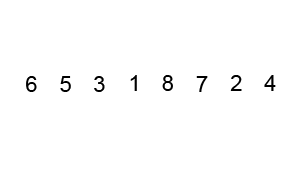# how to sort map values in go

Hi guys,
this is day 11 out of 100 days of go coding.

This time it is very short demo of go maps and simple sort by map values. Note that the example below will not work correctly if values has duplicates.

```package main

import (
"fmt"
"sort"
)

func main() {
//init a map with strings keys and int values
var m = make(map[string]int)
m["Sun"] = 7
m["Sat"] = 6
m["Fri"] = 5
m["Thu"] = 4
m["Mon"] = 1
m["Tue"] = 2
m["Wed"] = 3

//reverse keys and values of m map
var days = make(map[int]string)
//array of indexes for sorting
var daykeys = make([]int, len(m))

fmt.Print("Unsorted days of week\n")
counter := 0
for k, v := range m {
fmt.Printf("%s -> %d\n", k, v)
days[v] = k
daykeys[counter] = v
counter++
}
//sort indexes array here
sort.Ints(daykeys)

fmt.Print("Sorted days of week\n")
for _, v := range daykeys {
fmt.Printf("%s\n", days[v])
}
}
```

I believe this code has a lot of room for optimization, so if you know how please write me in comments or ping me in twitter with your version.

Happy coding everyone!